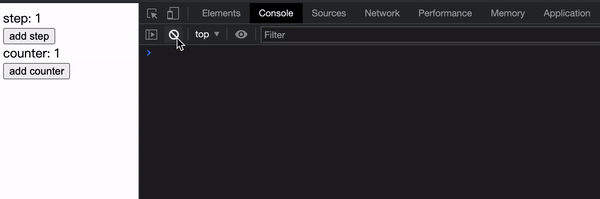# Model 通信#

Model 通信，既指不同 Model 间的通信，也指同一个 Model 内部 Effects、Actions 之间的通信。

## Model 间通信#

Model 之间不是孤立的，是可以进行通信的。主要分为两种场景：

1. 在 Model 中访问其它 Model 的 State 和 Actions。
2. 在 Model 中监听其它 Model 变化。

``````import { model } from '@modern-js/runtime/model';

const stepModel = model('step').define({
state: 1,
});

const counterModel = model('count').define((context, { use, onMount }) => {
const [, , subscribeStep] = use(stepModel);

onMount(() => {
return subscribeStep(() => {
console.log(
`Subscribe in counterModel: stepModel change to \${use(stepModel)}`,
);
});
});

return {
state: {
value: 1,
},
actions: {
const step = use(stepModel);
state.value += step;
},
},
};
});

export { stepModel, counterModel };``````

`stepModel` 只声明一个 `state`，初始值为 1。

`counterModel` 通过 `use` 函数加载 `stepModel`，拿到返回的 `subscribeStep` 函数，用来监听 `stepModel` 状态的变更。 `onMount` 是 Model 挂载完成后的钩子函数，`counterModel` 挂载完成后开始订阅 `stepModel` 状态的变更，打印出 `stepModel` 的最新值。

`counterModel` 通过 `use` 函数访问 `stepModel`，在 `add` 里可以获取到当前 `stepModel` 的值（步频），以此值来做自增。

``````import { useModel } from '@modern-js/runtime/model';
import { counterModel, stepModel } from './models/count';

function Counter() {
const [state, actions] = useModel(counterModel);
const [step, stepActions] = useModel(stepModel);

return (
<div>
<div>step: {step}</div>
<button onClick={() => stepActions.setState(step + 1)}>add step</button>
<div>counter: {state.value}</div>
</div>
);
}

export default function App() {
return <Counter />;
}``````

Modern.js 默认开启 自动生成 actions，所以 `stepModel` 中虽然没有手动定义 Actions，但可以使用自动生成的 `setState```````const barModel = model('bar').define({
// 省略
});

const fooModel = model('foo').define((context, utils) => {
// 获取 barModel 的 actions
const [, actions] = utils.use(barModel);
return {
// 省略 state、actions
effects: {
// 省略副作用逻辑
// 调用 barModel 的 action
barModel.actionA();
},
// 省略副作用逻辑
// 调用 barModel 的 action
barModel.actionB();
},
},
};
});``````

## Model 内通信#

Model 内通信，也主要分为两种场景：

1. Effects 函数调用自身 Model 的 Actions 函数、或其他 Effects 函数。
2. Actions 函数调用自身 Model 的 其他 Actions 函数。

``````const fooModel = model('foo').define((context, { use, onMount }) => ({
state: {
a: '',
b: '',
},
actions: {
},
},
},
effects: {
// 通过 use 获取当前 Model 的 actions
const [, actions] = use(fooModel);
const res = await mockFetchA();
actions.setA(res);
},
// 通过 use 获取当前 Model 的 actions
const [, actions] = use(fooModel);
const res = await mockFetchB();
actions.setB(res);
},
},
}));``````

``````const fooModel = model('foo').define((context, { use, onMount }) => {
let actions;

onMount(() => {
// fooModel 挂载完成后，通过 use 获取当前 Model 的 actions
[, actions] = use(fooModel);
});

return {
state: {
a: '',
b: '',
},
actions: {
},
},
},
effects: {
const res = await mockFetchA();
actions.setA(res);
},